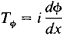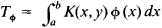[¦self ə¦jȯint ′äp·ə‚rād·ər]
(mathematics)
A linear operator which is identical with its adjoint operator.

(or Hermitian operator), an operator coincident with its adjoint. The theory of self-adjoint operators arose as a generalization of the theory of, for example, integral equations with a symmetric kernel, self-adjoint differential equations, and symmetric matrices. Examples of self-adjoint operators are (1) the operator of multiplication by the independent variable in the space of functions that are defined on the entire number axis and are square integrable and (2) the differentiation operatorin the same space.

If the function K(x,y) is continuous in the square a ≤ x ≤ b,a ≤ y ≤ b and if K(x,y) = K(y, x), then the integral operatoris self-adjoint. The spectrum of a self-adjoint operator lies on the real axis. In quantum mechanics physical quantities have corresponding self-adjoint operators whose spectra give the possible values of these quantities. A self-adjoint operator can be represented as an integral that is the limit of linear combinations of pairwise orthogonal projection operators with real coefficients.

References in periodicals archive ?
Then there exists a bounded selfadjoint operator A defined on H such that
These estimations will allow to study convergence and summability properties of spectral expansions connected with perturbed selfadjoint operator.
Such a selfadjoint operator S' [contains] S is called a self-adjoint extension of S.
It is known that [bar.T] is an injective closed symmetric operator, [[bar.T].sub.1] is a non injective selfadjoint operator, and [bar.T] [subset] [[bar.T].sub.1] [subset] [T.sup.*] (see pp.
The operator associated with this eigenvalue problem is not selfadjoint, but it can be symmetrized in the sense that one can prove the existence of a selfadjoint operator which has the same spectrum as the original operator.
where [Q.sub.1], [Q.sub.2] are strongly measurable operator valued functions taking values in the space of positive selfadjoint operators [L.sup.+.sub.s](E), and R taking values from [L.sup.+.sub.s](U) having continuous inverse.
Dragomir, Hermite-Hadamard's type inequalities for convex functions of selfadjoint operators in Hilbert spaces, Linear Algebra Appl., 436(2012), 1503-1515.
The spectral behaviour of these operators exhibits many new phenomena compared to selfadjoint operators. The spectral theorem and variational principles are not valid.
Do quantum mechanics, represented by selfadjoint operators in Hilbert space contradict classical (in our case) Hamiltonian mechanics represented by Borel functions in phase space?
Berezans'kii, Expansions in eigenfunctions of selfadjoint operators, Translated from the Russian by R.
The book begins by reviewing the necessary background on selfadjoint operators, semigroups, Kolmogorov diffusion processes, and solutions of stochastic differential equations.
Notice also that the operators [[??].sub.1](s) and [[??].sub.2] (s) are selfadjoint operators.

Site: Follow: Share:
Open / Close